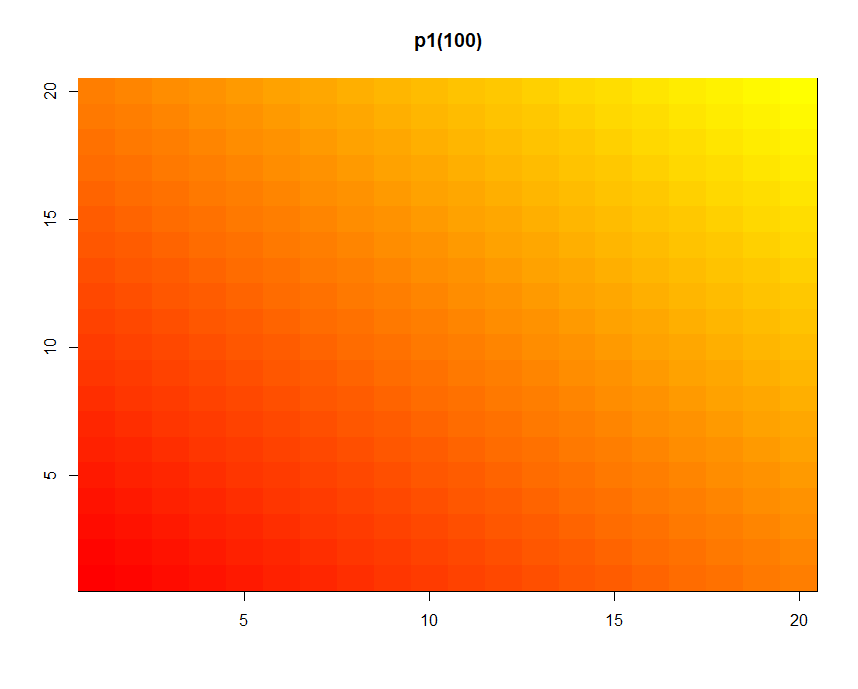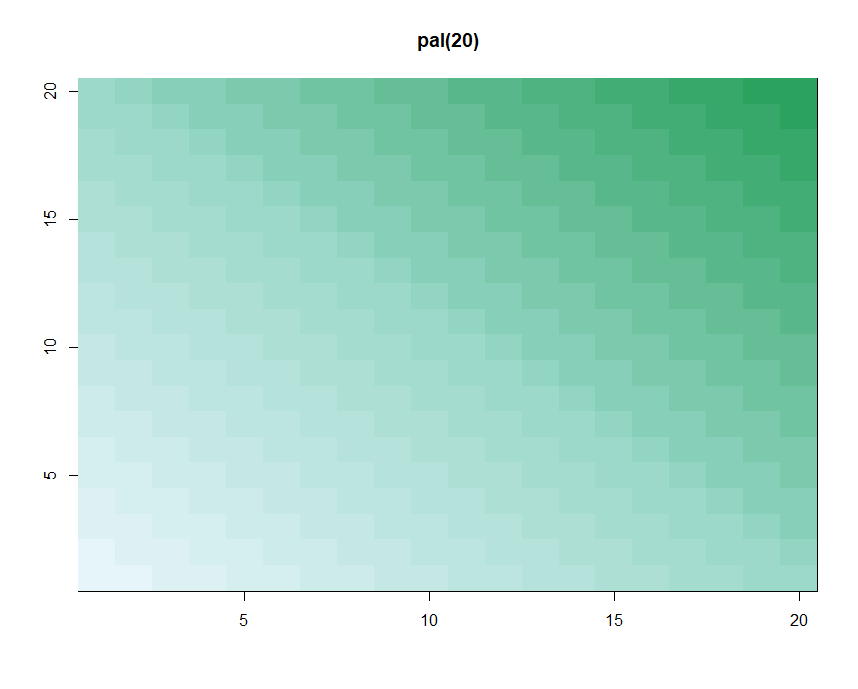0%

# Princlples of Analytic Graphics

1. Show comparisons compared to what(PM25 in house with aircleaner compared to without aircleaner)
2. Show casuality, mechanism, explanation show how you believe the world works(show you believe child living in house with lower pm25 is more likely to be healthy)
3. Show multivariate Data more than 2 variables
4. Integrate multiple models of evidence don't let the tools drive the analysis(plot depend on your own idea, not the tools)
5. Describe and document the evidence
6. Content is king

# Take a Look at the Data

1. Summary
2. Boxplots
3. Historgrams
4. Barplot

## Two dimensions

1. Multiple Boxplots
2. Multiple Historgrams
3. Scatterplot

# Plotting Systems in R

## Base Plotting System

• Base:artist's palette model, and usually needs two steps to create a plot
• representative:plot()

### Two packages

• graphics(including plot, hist, boxplot, etc)
• grDevices(including X11, PDF, PostScript, PNG, etc)

### Two steps to create a base plot

• Initializing a new plot
• Annotation an existing plot

### Base Plotting Functions

#### Initialize

• plot:initialize a new plot
• hist:initialize a new hist
• boxplot:initialize a new boxplot

• lines:add lines to a plot
• abline:add lines to a plot
• points:add points to a plot
• text:add text labels to a plot using specified x, y coordinates
• title:add annotations to x, y axis labels, title, subtitle, outer margin
• mtext:add arbitrary text to the margins

### Some Important Base Graphics Parameters

• pch:the plotting symbol
• lty:the line type
• lwd:the line width
• col:color
• xlab:string for the xlab
• ylab:string for the ylab
• las:the orientation of the axis
• bg:thebackground color
• mar:the margin size
• oma:the outer margin size
• mfrow:number of plots per row, column
• mfcol:number of plots per row, column(differ in order)

Default parameters:

### Examples

#### Example:Legend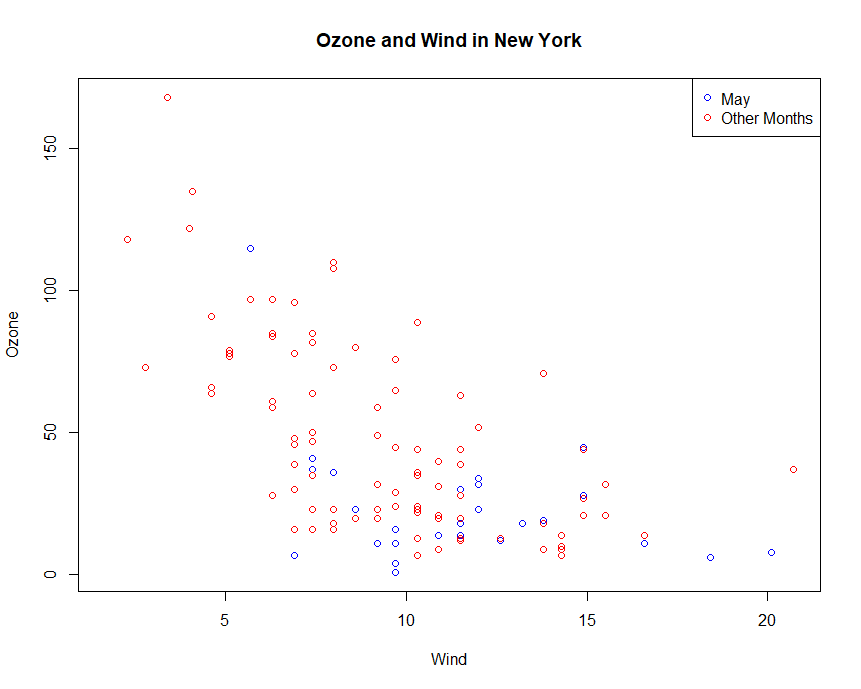#### Example:Multiple Base Plots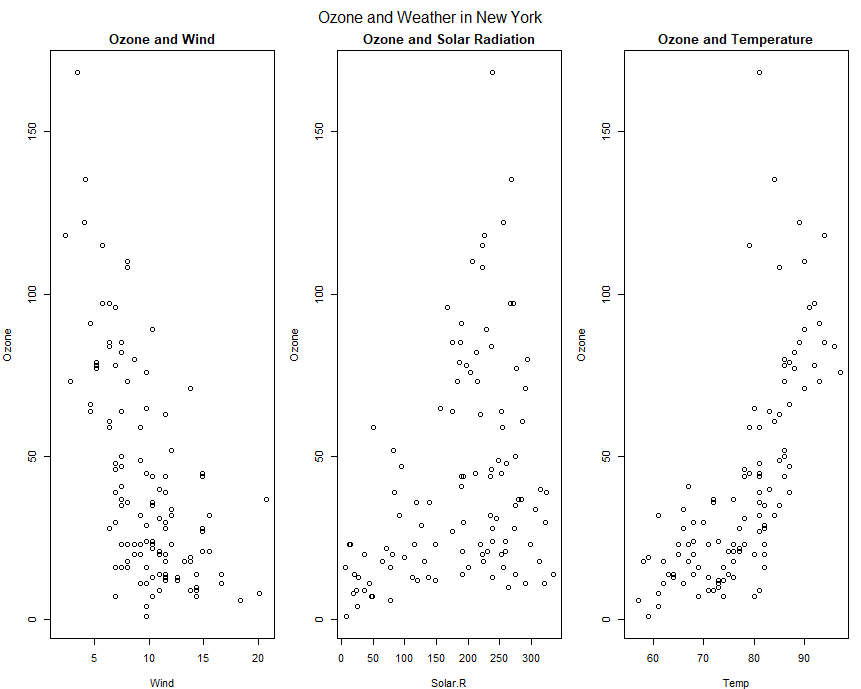## The Lattice System

• Lattice:Entire plot specified by one function
• useful for plotting high dimensional data(conditioning plots)
• different from base plot driectly to the graphics device, lattice plot returns an object of class trellis (and will be auto-printed)
• representative:xyplot()

### Two packages

• lattice(including xyplot bwplot, levelplot, etc)
• grid(usually indirectedly called through lattice or ggplot2)

### Lattice Functions

• xplot:create scatterplots
• bwplot:box-and-whiskers plots
• histogram:histograms
• stripplot:like a boxplot but with actual points
• dotplot:plot dots on "violin strings"
• splom:scatterplot matrix(like pairs in base plotting)
• levelplot, contourplot:for plotting "image" data

### Examples

#### Example:xyplot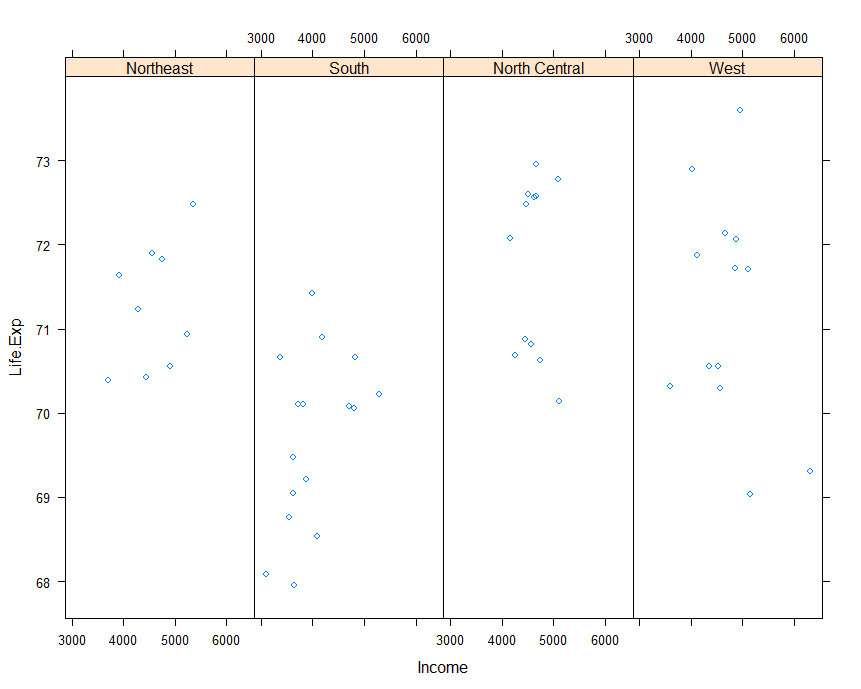#### Example:plane functiuon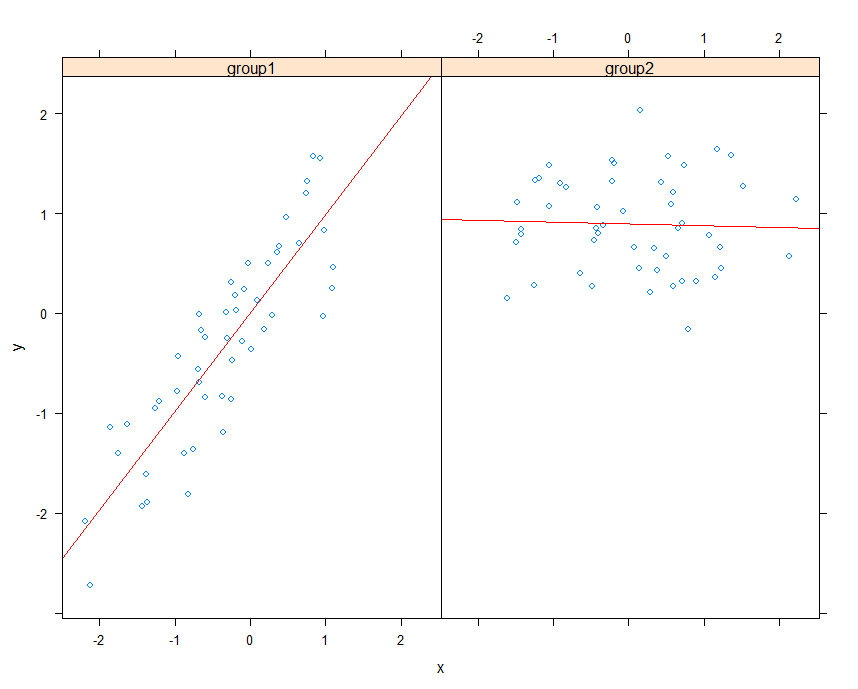## The ggplot2 System

• ggplot2:Mixed elements of Base and Lattice
• book: In brief, thegrammar tells us that a statistical graphic is a mapping from data to aesthetic(美学) attributes(color, shape, size) of geometric objects (points, lines, bars).The plot may also contain statistical transformations of the data and is drawn on a specific corrdinate system.
• representative:qplot(), ggplot()

### Basic Components of a ggplot2 Plot

• a data frame
• aesthetic mapping:how data are mapped to color, size
• geoms:points, lines, shapes
• facets:for conditional plots
• stats:binning(柱形分析), quantiles, smoothing
• scales:for example:sex
• corrdinate system

### Annotation

1. labs and theme
• xlab(), ylab(), ggtitle(), labs()
• theme(legend.position = "none")
• theme_gray()
• theme_bw()

2. ylim and coord_cartesian - ylim(-3, 3)# 把y限定在(-3, 3) - coord_cartesian(ylim = c(-3, 3))# 显示(-3, 3)的范围

3. cut

### Examples

#### Example:geom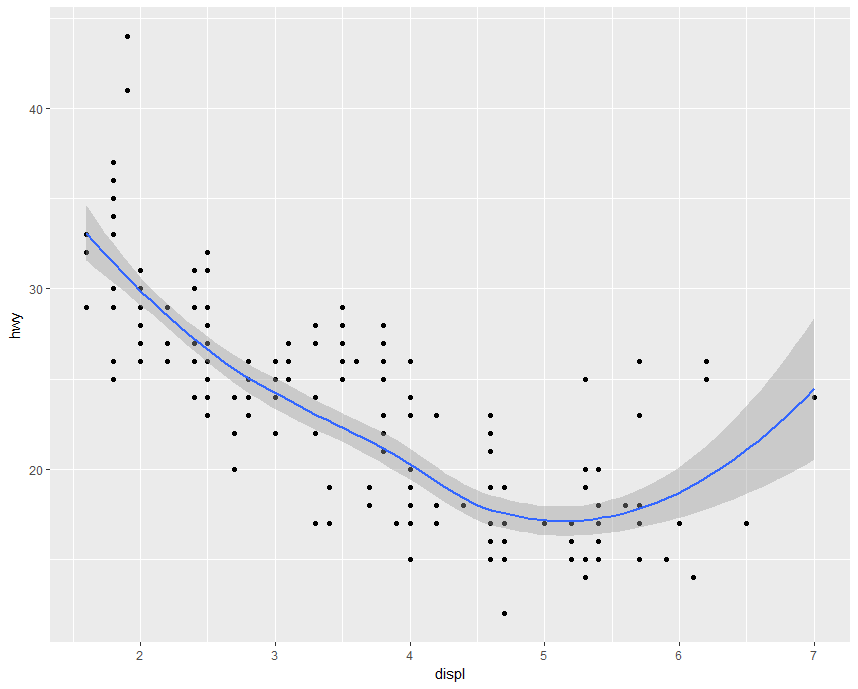#### Example:fill#### Example:facets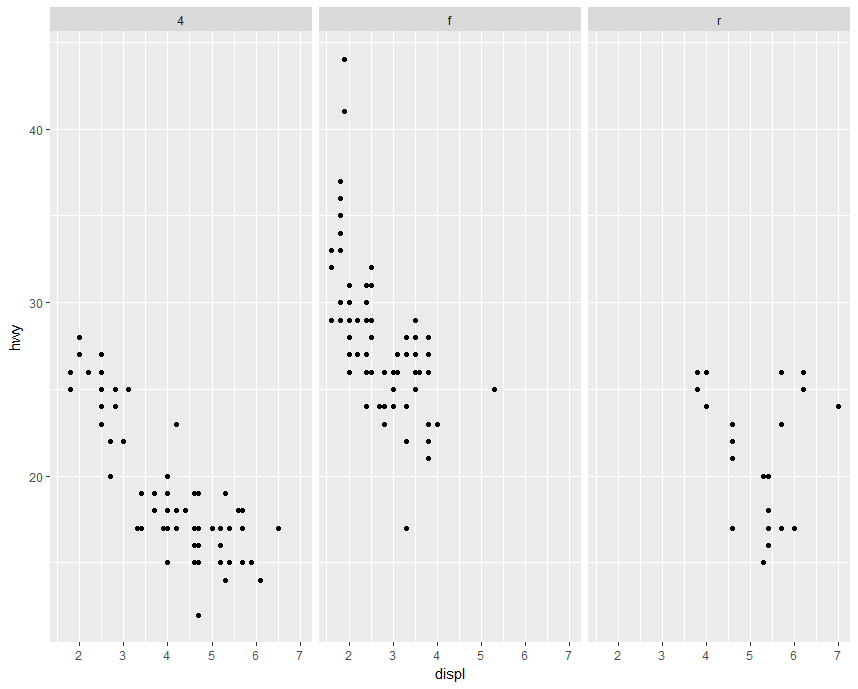#### Example:boxplot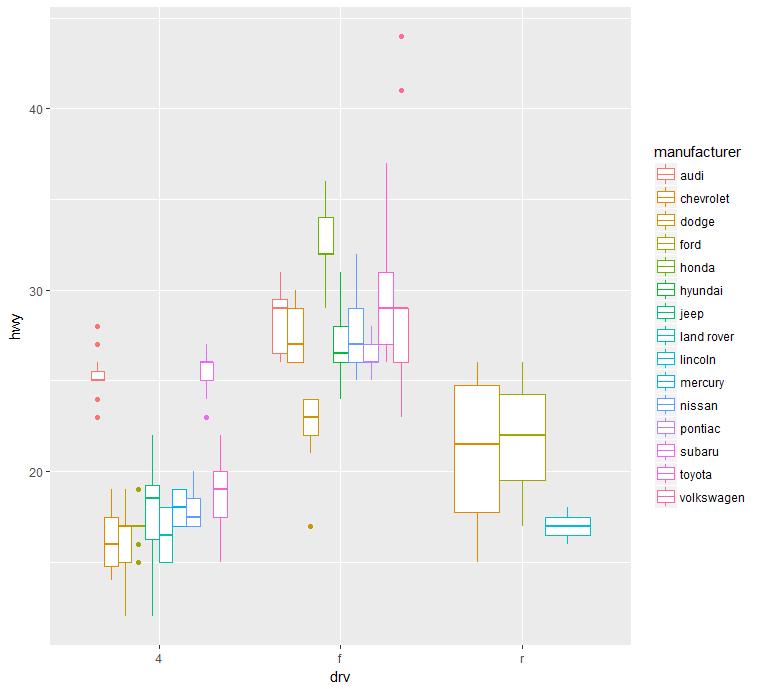#### Example:ggplot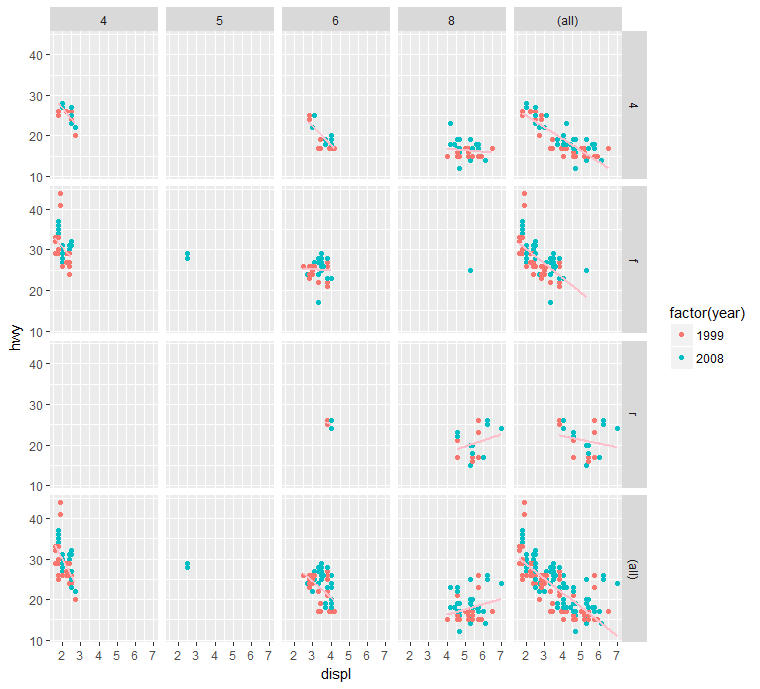# Color